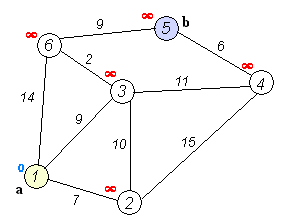# Dijkstra’s Algorithm

Sometime back I talked about Graph data structure.

One of the important usage of graph is to find the shortest path from one node to another, for example in travelling salesman problem.

One important algorithm to find shortest path for between two nodes in graph is Dijkstra’s algo.

Here is howImage source wikipedia

1. Mark All node distance as infinity (to mark that node has not been traversed yet)
2. Start from source node, add all other nodes to a set contaning unvisited node
3. Traverse all neighboring nodes from current node N and calculate distance from previous node by adding distance[N] + distance from N to this node (edge weight)
4. When all neighbors are calculated, mark current node as visited.
5. If we have visited the destination node, exit with the distance till destination node.
5. Pull next node from set.
6. Goto 3.

Read more here